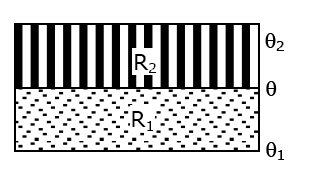The temperature θ at the junction of two insulating sheets, having thermal resistances R1 and R2

The temperature θ at the junction of two insulating sheets, having thermal resistances R1 and R2 as well as the bottom and top temperatures θ1 and θ2 (as shown in the figure) is given by:a. (θ1R2 + θ2R1)/(R1 + R2)

b. (θ1R2 – θ2R1)/(R2 – R1)

c. (θ2R2 – θ1R1)/(R2 – R1)

d. (θ1R1 + θ2R2)/(R1 + R2)

Temperature at the junction is θ.

So, using the formula,

(T2 – T)/R2 = (T – T1)/R1

2 – θ)/R2 = (θ – θ1)/R1

R12 – θ) = R2(θ – θ1)

R1θ2 – R1θ = R2θ – R2θ1

R1θ + R2θ = R1θ2 + R2θ1

θ = (R1θ2 + R2θ1)/(R1+R2)(0)(0)